### Home > PC3 > Chapter 8 > Lesson 8.3.3 > Problem8-122

8-122.
1. Angles u and v are in the same quadrant. If cos(u) =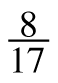and sin(v) = –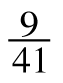, evaluate: Homework Help ✎

1. tan(u + v)

2. cos(vu)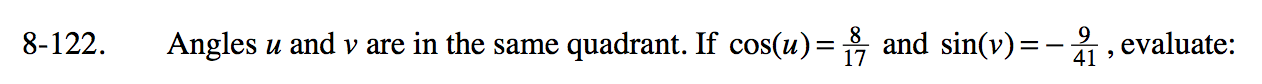If cosine is positive and sine is negative, which quadrant are u and v in?

Draw a triangle diagram for each angle. One is done for you.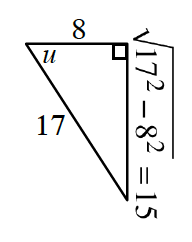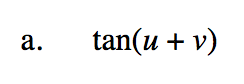$\frac{\tan(u)+\tan(v)}{1-\tan(u)\tan(v)}$

$\frac{\left(\frac{-15}{8}\right)+\tan(v)}{1-\left(\frac{-15}{8}\right)\tan(v)}$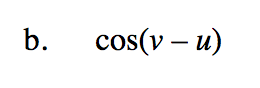$\cos(v)\cos(u)+\sin(v)\sin(u)$

$\cos(v)\left(\frac{8}{17}\right)+\sin(v)\left(\frac{-15}{17}\right)$